### IMO Shortlist 2009 problem N2

Kvaliteta:
Avg: 4.0
Težina:
Avg: 6.0
A positive integer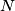$N$ is called balanced, if$N=1$ or if$N$ can be written as a product of an even number of not necessarily distinct primes. Given positive integers$a$ and$b$, consider the polynomial$P$ defined by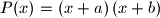$P\!\left(x\right) = \left(x+a\right)\left(x+b\right)$.
a) Prove that there exist distinct positive integers$a$ and$b$ such that all the number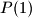$P\!\left(1\right)$,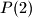$P\!\left(2\right)$, ...,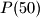$P\!\left(50\right)$ are balanced.
b) Prove that if$P\!\left(n\right)$ is balanced for all positive integers$n$, then$a=b$.

Proposed by Jorge Tipe, Peru
Source: Međunarodna matematička olimpijada, shortlist 2009